Function Repository Resource:

# DiscreteDolphChebyshevWindow

Return a discrete Dolph-Chebyshev window sequence

Contributed by: Mariusz Jankowski
 ResourceFunction["DiscreteDolphChebyshevWindow"][n] gives the discrete Dolph-Chebyshev window of length n. ResourceFunction["DiscreteDolphChebyshevWindow"][n,α] gives the discrete window with spectral side lobe attenuation of -20α dB.

## Details and Options

The argument n should be an Integer defining the length of the window.
The argument α is a positive number, defining the spectral side lobe attenuation of the window. The default value is α=2.

## Examples

### Basic Examples (2)

A Dolph-Chebyshev window of length 21:

 In:=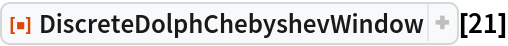Out=Plot the window:

 In:=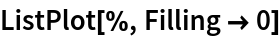Out=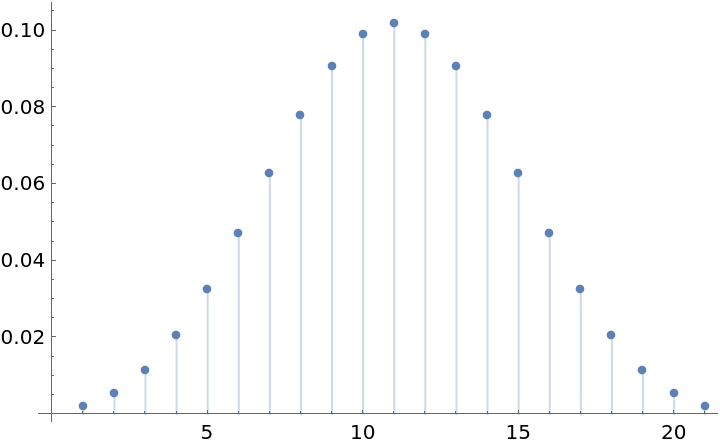A Dolph-Chebyshev window with a specified parameter:

 In:=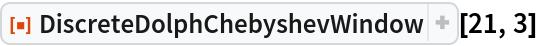Out=### Applications (2)

Create a lowpass FIR filter with cut-off frequency of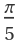and length 15:

 In:=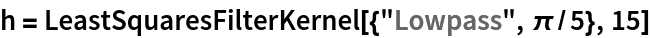Out=Apply a Dolph-Chebyshev window to the filter to improve stop-band attenuation:

 In:=Log-magnitude plot of the power spectra of the two filters:

 In:=Out=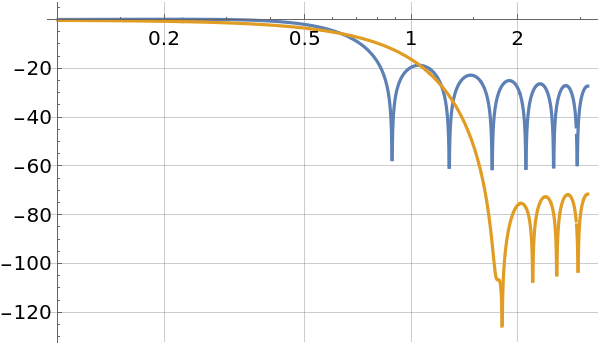Use the Dolph-Chebyshev window to diminish the effect of signal partitioning when computing a spectrogram:

 In:=Out=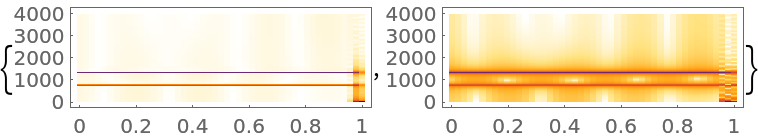### Properties and Relations (2)

The Dolph-Chebyshev window side lobes are equiripple:

 In:=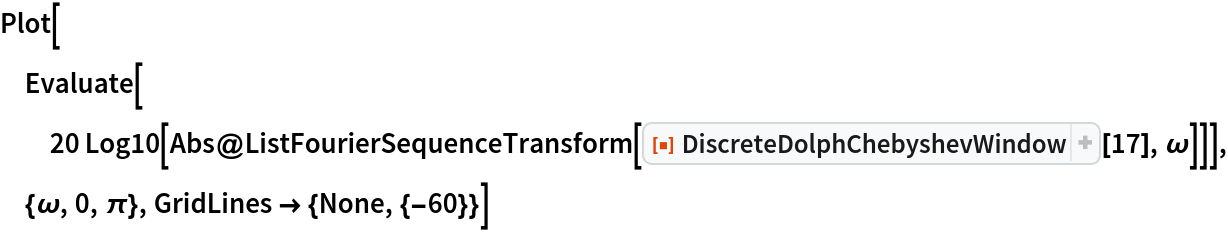Out=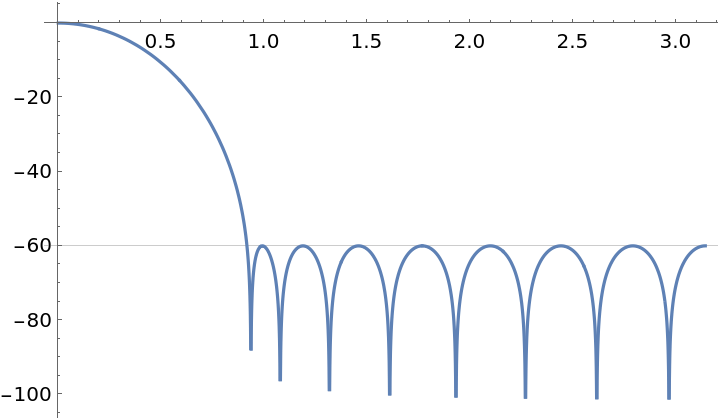The Dolph-Chebyshev window side lobe attenuation is equal to -20α dB:

 In:=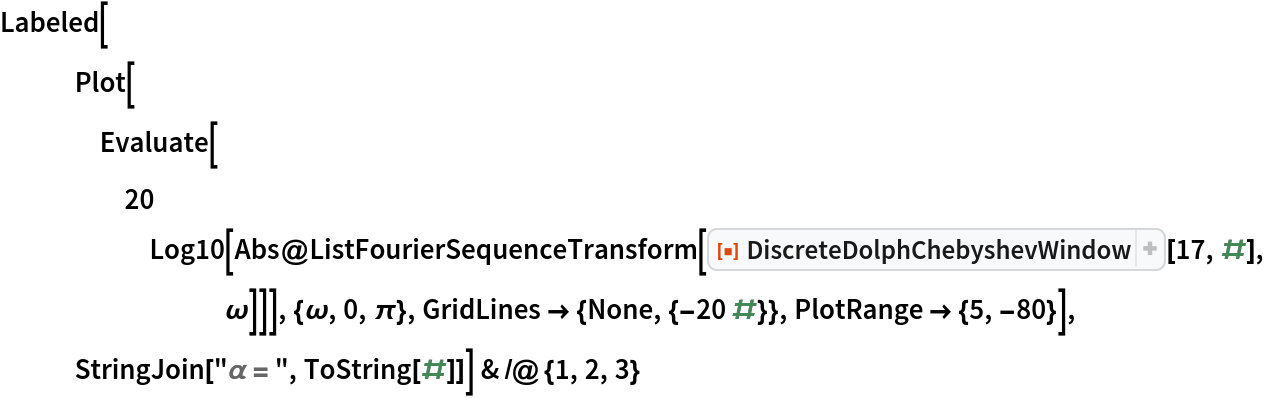Out=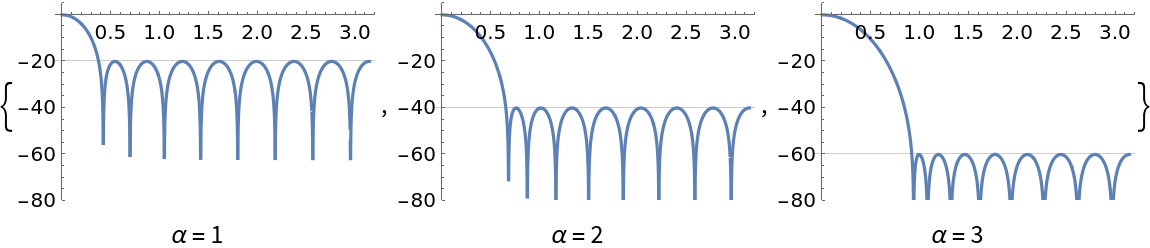### Possible Issues (1)

For small α, the window end-points may be larger than other sample values. The magnitude of the end-point impulses is on the order of the size of the spectral side lobes:

 In:=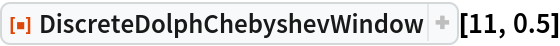Out=In:=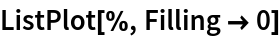Out=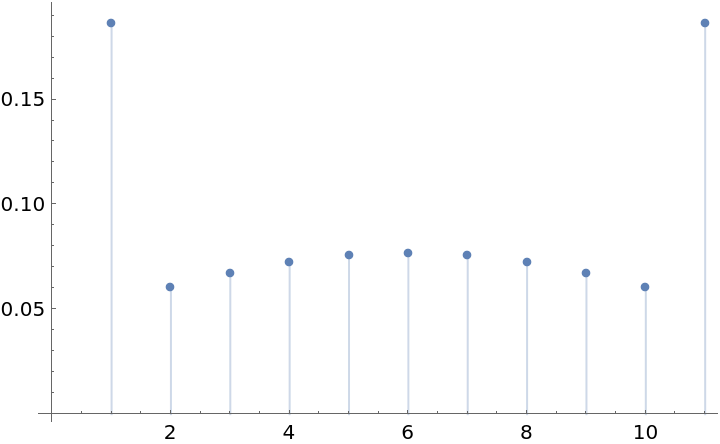## Publisher

Mariusz Jankowski

## Version History

• 1.0.0 – 08 July 2020

NA